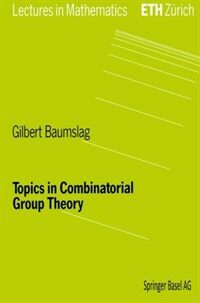> 상세정보

# 상세정보## Topics in combinatorial group theory (1회 대출)

자료유형
단행본
개인저자
Baumslag, Gilbert.
서명 / 저자사항
Topics in combinatorial group theory / Gilbert Baumslag.
발행사항
Basel ;   Boston :   Birkhauser,   1993.
형태사항
vi, 164 p. : ill. ; 24 cm.
총서사항
Lectures in mathematics ETH Zurich
ISBN
3764329211 (Basel : acid-free paper) : 0817629211 (Boston : acid-free paper)
일반주기
Includes index.
일반주제명
Combinatorial group theory.
 000 00780namuu2200229 a 4500 001 000001060021 005 20010611113333 008 930604s1993 sz a 001 0 eng 020 ▼a 3764329211 (Basel : acid-free paper) : ▼c 34.00F (est.) 020 ▼a 0817629211 (Boston : acid-free paper) 040 ▼a DLC ▼c DLC ▼d DLC ▼d 244002 049 0 ▼l 151101229 050 0 0 ▼a QA182.5 ▼b .B38 1993 082 0 0 ▼a 512/.2 ▼2 20 090 ▼a 512.2 ▼b B348t 100 1 ▼a Baumslag, Gilbert. 245 1 0 ▼a Topics in combinatorial group theory / ▼c Gilbert Baumslag. 260 ▼a Basel ; ▼a Boston : ▼b Birkhauser, ▼c 1993. 300 ▼a vi, 164 p. : ▼b ill. ; ▼c 24 cm. 440 0 ▼a Lectures in mathematics ETH Zurich 500 ▼a Includes index. 650 0 ▼a Combinatorial group theory.

### 소장정보

No. 소장처 청구기호 등록번호 도서상태 반납예정일 예약 서비스
No. 1 소장처 청구기호 512.2 B348t 등록번호 151101229 도서상태 대출중 반납예정일 2030-12-31 예약 서비스

### 컨텐츠정보

#### 목차

```CONTENTS
Preface = Ⅶ
Acknowledgements = Ⅶ
ChapterⅠ History
1. Introduction = 1
2. The beginnings = 1
3. Finitely presented groups = 2
4. More history = 5
5. Higman's marvellous theorem = 8
6. Varieties of groups = 9
7. Small Cancellation Theory = 14
Chapter Ⅱ The Weak Burnside Problem
1. Introduction = 17
2. The Grigorchuk-Gupta-Sidki groups = 19
3. An application to associative algebras = 27
Chapter Ⅲ Free groups, the calculus of presentations and the method of Reidemeister and Schreier
1. Frobenius' representation = 29
2. Semidirect products = 33
3. Subgroups of free groups are free = 37
4. The calculus of presentations = 47
5. The calculus of presentations(continued) = 49
6. The Reidemeister-Schreier method = 55
7. Generalized free products = 58
Chapter Ⅳ Recursively presented groups, word problems and some applications of the Reidemeister-Schreier method
1. Recursively presented groups = 61
2. Some word problems = 63
3. Groups with free subgroups = 64
Chapter Ⅴ Affine algebraic sets and the representative theory of finitely generated groups
1. Background = 75
2. Some basic algebraic geometry = 76
3. More basic algebraic geometry = 80
4. Useful notions from topology = 82
5. Morphisms = 85
6. Dimension = 90
7. Representations of the free group of rank two in SL(2, C) = 93
8. Affine algebraic sets of characters = 99
Chapter Ⅵ Generalized free products and HNN extensions
1. Applications = 103
2. Back to basics = 107
3. More applicatons = 111
4. Some word, conjugacy and isomorphism problems = 120
Chapter Ⅶ Groups acting on trees
1. Basic definitions = 123
2. Covering space theory = 129
3. Graphs of groups = 131
4. Trees = 134
5. The fundamental group of a graph of groups = 137
6. The fundamental group of a graph of groups(continued) = 139
7. Group actions and graphs of groups = 143
8. Universal covers = 147
9. The proof of Theorem 2 = 153
10. Some consequences of Theorem 2 and 3 = 154
11. The tree of SL2 = 158
Index = 163```

### 관련분야 신착자료

#### Algebra

Aluffi, Paolo (2021)

김대수 (2022)

민만식 (2022)